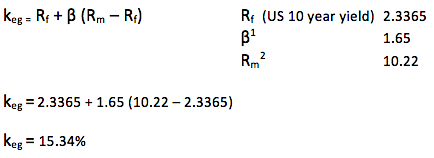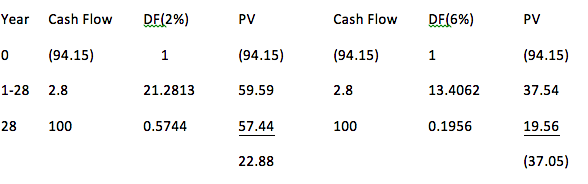# How To Apply WACC: Weighted Average Cost Of Capital

In previous articles, using Caterpillar, Inc. (CAT) for the figures, we have looked at different ways in which to value the company. Those considered so far have been Asset Valuation, Calculated Intangible Value and P/E Method. In a future article we will consider perhaps the most reliable – the discounted cash flow. To calculate this, we need a weighted average cost of capital (WACC) for CAT, and that is what we will examine in this article.

The WACC is the average cost of the company’s finance; this will include equity, preference shares, bank loans and bonds. It is generally accepted that the WACC will be used to appraise long term investments; therefore it is most appropriate to include only long term debt.

The formula for WACC is:

ko = keg (Ve/Ve +Vd) + kd (Vd/ Ve +Vd)

The first step is to calculate the Keg (cost of equity), using the Capital Asset Pricing Model:Next, we must identify the (Ve )3 market value of equity:

605.4 million shares x \$102.09 (current share price) = \$62,296 million

Market value of debt (Vd)4:

At 30/09/14, long term debt of \$28,180 million. You could choose to use total liabilities but I prefer to use LT debt, as mentioned in the opening paragraph.

As we will see later, the bonds are currently trading at \$94.15, therefore the market value of the debt is 94.15/100 x 28,180 = \$26,531 million

Cost of debt (kd)

CAT has issued debt5 which will mature in 2042, the interest rate was 3.803%. We have to calculate the net of tax payments (CAT’s viewpoint) and also calculate the kd for these redeemable bonds

Effective tax rate at 30/09/14 was 26.36%6

Interest payments are then 3.803 x (1-.2636) = 2.8%.

We can now estimate the Internal Rate of Return by calculating two NPVs:*Note that the cash flow in year 0 is current market value of the bond7

The IRR is:

L + (NPVL / NPVL-NPVH) (H-L)

2 + (22.88 / 22.88+37.05) (6-2)

2 + (0.38) (4)

3.52% is the cost of debt

We can now calculate the WACC:

ko = keg (Ve/Ve +Vd) + kd (Vd/ Ve +Vd)

15.34 (62,296 / 62,296 + 26,351) + 3.52 (26,351 / 62,296 + 26,351)

15.34 (0.7) + 3.52 (0.3)

10.74 + 1.06

WACC is 11.8%

In my next article, we will use this number to calculate a valuation for CAT based on discounted cash flows. Thanks for reading.

References: